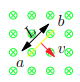# Problem: ± Motional EMF in a Conducting RodIn the figure, a conducting rod with length L = 25.0 cm moves in a magnetic field B of magnitude 0.500 T directed into the plane of the figure. The rod moves with speed v = 7.00 m/s in the direction shown. (Figure 1)(a) When the charges in the rod are in equilibrium, which point, a or b, has an excess of positive charge? (b) In what direction does the electric field then point?(c) When the charges in the rod are in equilibrium, what are the magnitude and direction of the electric field within the rod? Express your answer in volts per meter to at least three significant digits.(d) Which point, a or b, is at higher potential?(e) What is the magnitude Vba of the potential difference between the ends of the rod?Express your answer in volts to at least three significant digits.(f) What is the magnitude ε of the motional emf induced in the rod?Express your answer in volts to at least three significant digits.(g) What is the potential difference across the rod if it moves (i) parallel to ab and (ii) directly out of the page?

###### FREE Expert Solution

(a) We'll determine the direction of the current using the right-hand rule - generator rule.

The direction of the magnetic force on a positive moving charge: Thumb - Velocity, Fingers - magnetic field, and Perpendicular to the palm - magnetic force.

Positive charges are pushed/pulled towards (b) or positive charges move towards (b). This also implies that negative charges move towards (a)

82% (477 ratings)###### Problem Details

± Motional EMF in a Conducting Rod
In the figure, a conducting rod with length L = 25.0 cm moves in a magnetic field B of magnitude 0.500 T directed into the plane of the figure. The rod moves with speed v = 7.00 m/s in the direction shown. (Figure 1)(a) When the charges in the rod are in equilibrium, which point, a or b, has an excess of positive charge?

(b) In what direction does the electric field then point?

(c) When the charges in the rod are in equilibrium, what are the magnitude and direction of the electric field within the rod?

Express your answer in volts per meter to at least three significant digits.

(d) Which point, a or b, is at higher potential?

(e) What is the magnitude Vba of the potential difference between the ends of the rod?